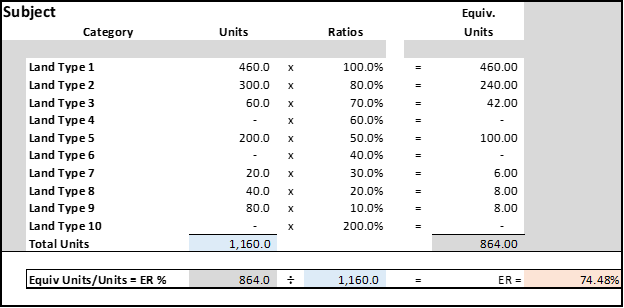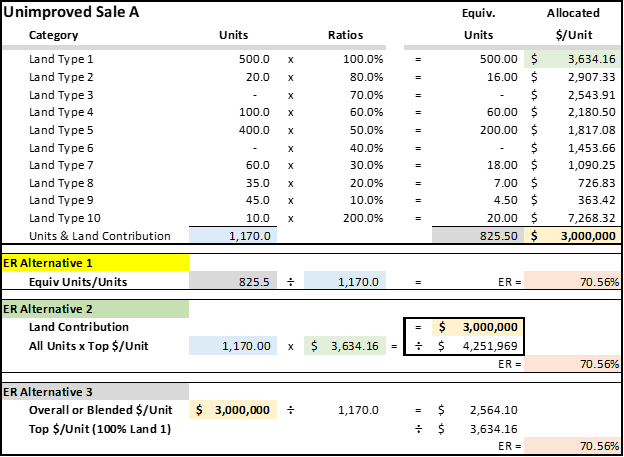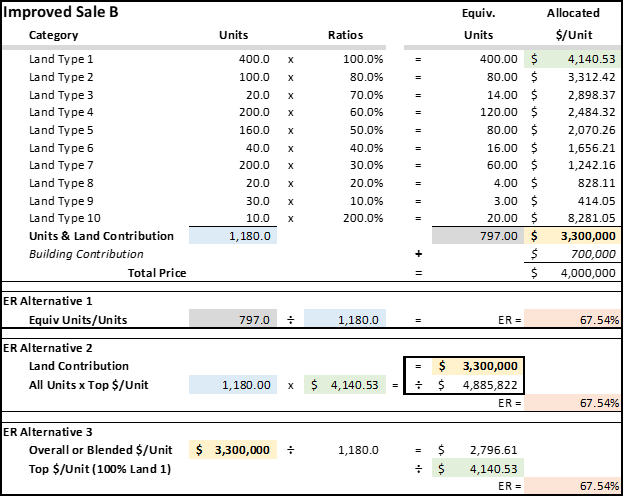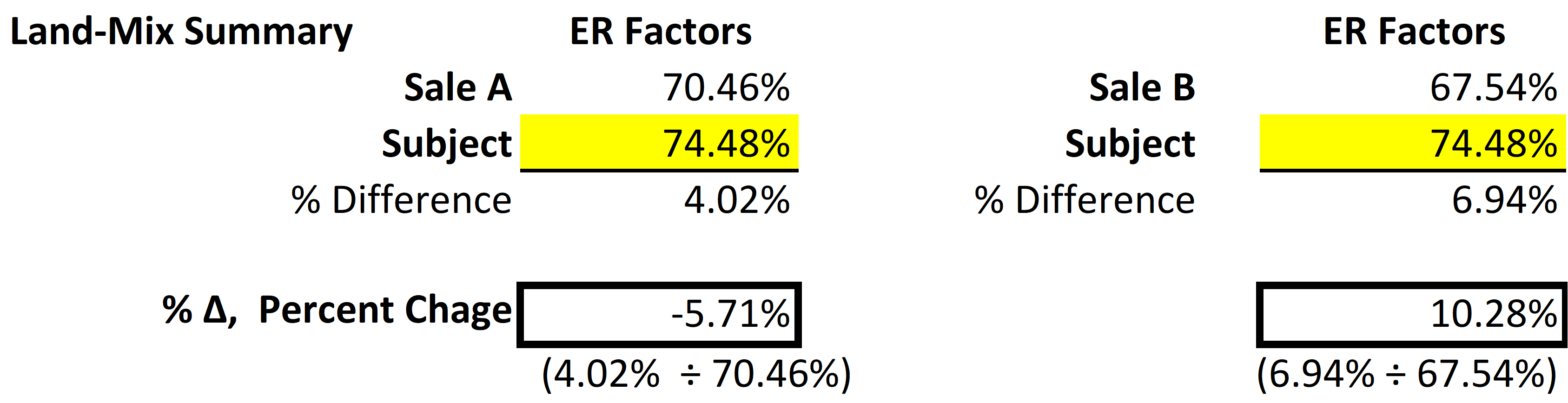The ER (equivalency rating) is a mathematical expression of each sale's land-mix or composition.  The ER replaces the ASFMRA’s traditional “land-mix” procedure that contained multiple computations.  The process converts the “land contribution” within each sale to a mathematical percentage, an expression of the “whole”.  To begin ER, subject ratios are required to express its mathematical composition (74.48% below).

The graphics below are:

1. Subject's equivalent units and ER calculated from ratios
2. Then, one (1) unimproved Sale and
3. one (1) improved Sale were reduced to the same mathematical “expression” or Equivalency Ratio to ultimately compute the “land-mix” adjustment for each.When this process is repeated for each sale, the percent change between the sales and subject provide the basis for a simple calculation for the differences in “land-mix” or composition disparities. Two sales are shown below, i.e., one unimproved and one improved; plus, three (3) ways to compute the Sale ER’s.

Note for Alternative 2 below:  The \$3,000,00 “Land Contribution” is divided by the “Gross Units x Top \$/unit” or 1,170 gross units x \$3,634.16/acre for Land Type 1 = \$4,251,969 which becomes the denominator for the calculation shown inside the smaller box (\$3,000,000  ÷ \$4,251,969 = 70.56%).The land-mix calculations are shown below:The % change for each sale is multiplied by its corresponding \$/acre to calculate the proper land-mix adjustment by comparison to the Subject.

NOTE:  If the Subject is improved, an additional adjustment may be required — to both sales.  Even though Sale B is improved, the structures may be different sizes, condition, and remaining effective ages and require additional consideration(s), or “+, -, or =” calculations for its final impact on the final price per acre.